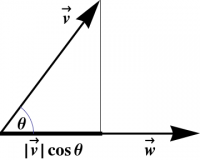Prerequisites

### The Dot Product

Before you read this section, you should take a few minutes to jot down everything you know about the dot product. Many students feel that if they know one representation for a concept, that is sufficient. As you will discover throughout this book, the professional scientist's ability to solve problems often comes from the ability to play off several different representations against each other.

The geometric definition of the dot product is given by: \begin{equation} \vv\cdot\ww = {|\vv| |\ww| \cos\theta} \label{DotGeom} \end{equation} where $\theta$ is the angle between $\vv$ and $\ww$. Now we notice something interesting: If $\vv$ is the vector along the hypotenuse of the triangle in Figure 1, then $|\vv| \cos\theta$ is the projection of $\vv$ onto the direction of $\ww$, the other vector. Similarly, $|\ww| \cos\theta$ is the projection of $\ww$ onto the direction $\vv$, so the dot product is a symmetrized version of projection.Figure 1: A projection can be found using the geometric definition of the dot product.

More precisely, in Figure 1, the length of the heavy line segment represents the component of $\vv$ in the $\ww$ direction. If we regard this component as the definition of the dot product of $\vv$ with the unit vector $\ww\over|\ww|$, then multiplying through by $|\ww|$ and using some triangle trigonometry leads immediately to equation ($\ref{DotGeom}$) for the dot product of $\vv$ and $\ww$. (Projecting $\ww$ onto $\vv$ instead leads to the same result.)

Thus, the dot product is a rescaled projection; it is the rescaling that makes it symmetric; it doesn't matter which vector is which.

Since the angle between a vector and itself is zero, an immediate consequence of this formula is that the dot product of a vector with itself gives the square of its magnitude, that is \begin{equation} \vv\cdot\vv = |\vv|^2 \end{equation} In particular, taking the “square” of any unit vector yields 1, for example \begin{equation} \xhat\cdot\xhat = 1 \end{equation} Furthermore, since $\cos{\pi\over2}=0$, it follows immediately from the geometric definition that two (nonzero) vectors are orthogonal if and only if their dot product vanishes, that is 1) \begin{equation} \vv\perp\ww \Longleftrightarrow \vv\cdot\ww = 0 \end{equation}

Because the dot product is distributive (i.e. you can “FOIL” the dot product over a sum of vectors), 2) it is easy to see that the geometric formula ($\ref{DotGeom}$) reduces to the standard component form of the dot product. For example, if $\vv=v_x\,\xhat+v_y\,\yhat$ and $\ww=w_x\,\xhat+w_y\,\yhat$, then \begin{eqnarray} \vv\cdot\ww &=& (v_x\,\xhat+v_y\,\yhat)\cdot(w_x\,\xhat+w_y\,\yhat) \nonumber\\ &=& v_x w_x \,\xhat\cdot\xhat + v_y w_y \,\yhat\cdot\yhat + v_x w_y \,\xhat\cdot\yhat + v_y w_x \,\yhat\cdot\xhat \nonumber\\ &=& v_x w_x + v_y w_y \end{eqnarray} This computation clearly works for any orthonormal basis. A special case is the dot product of a vector with itself, which reduces to the Pythagorean theorem when written out in terms of components, for example \begin{equation} \vv\cdot\vv = |\vv|^2 = v_x^2 + v_y^2 \end{equation}

In the above computation we simply “multiplied out” the original expression in terms of components, then used the multiplication table \begin{eqnarray} \xhat\cdot\xhat = 1 = \yhat\cdot\yhat\\ \end{eqnarray} \begin{eqnarray} \xhat\cdot\yhat = 0 = \yhat\cdot\xhat \end{eqnarray} Using the multiplication table of basis vectors under the dot product in this way is a useful strategy which you are encouraged to practice.

Returning to our earlier example of motion at 3 mph to the east and 4 mph to the north, we have $\uu=3\,\xhat+4\,\yhat$, so that \begin{eqnarray} |\uu|^2 = \uu\cdot\uu = 3^2 + 4^2 = 5^2 \end{eqnarray} and the overall speed is $|\uu|=5 \hbox{mph}$ as expected.

A good test of your understanding of the dot product is to try to use it to find the angle between the diagonal of a cube and one of its edges, or the angle between two diagonals.

1) Since the zero vector $\zero$ also satisfies this condition, it is, by definition, orthogonal to every other vector!
2) There is some fine print here: we must show that the dot product is, in fact, linear in its arguments, so that it distributes over addition as claimed. This property can be established geometrically; see our online article at this URL.

##### Views